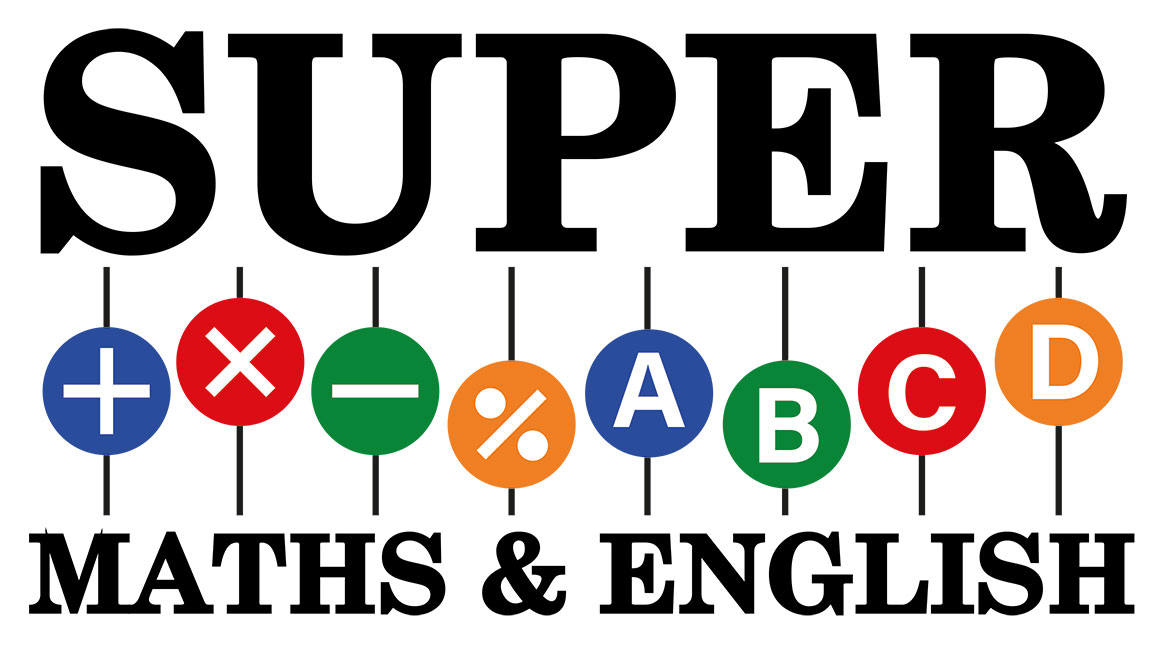SupermathsTeachers help develop a healthy interest in students by making learning fun and easy. We have well-trained Abacus Maths tutors who firstly understand the varied needs of the students. Secondly, develop a bond with them and then slowly introduce the concept of the Abacus to them.

##### Training

At Supermaths, we also train our Abacus Maths tutors to handle different kinds of students. We believe that every kid, even if he is dyslexic or dyscalculia should learn to handle the Abacus. So that they can use the Abacus tool as a means of doing mathematical calculations. Some of the kids have difficulty in understanding the different procedures involved in solving Maths problems. Our Abacus maths tutors devote extra time to those students so they can overcome this inability.

We teach the students enrolling with us, to use the Abacus in three different levels. For all students learning to handle the Abacus makes for the first level. Our Abacus maths tutors help students to learn and understand the Abacus. It consists of two parts:

• An upper “heavenly” region consisting of 1 bead in each vertical road
• A lower “earthly” region with each rod consisting of 4 beads

For the convenience of the students, our Abacus Maths tutors teach them to think of the beads as numbers.

The upper beads hold the value 5 each. The lower beads value differently with the beads on the extreme right column holding the value at 1. We, at Supermaths, slowly and repeatedly make the students practice with the beads. We also acquaint them with the changing values of the lower beads as one goes from right to left.

The three techniques

At Supermaths, we follow a rather unique program.  In this program, our Abacus maths tutors initially teach the students to do mathematical calculations using the physical tool. Finger technique follows it and finally comes the mental technique.

In the finger technique, we teach kids to use their fingers to handle the Abacus in a way that they perform the calculations with only a few fingers.

Students learn the mental technique in the last levels. Abacus maths tutors teaching this level in our Abacus maths institute, Supermaths, get special trainings. They get trained to help students visualize and imagine the Abacus in their minds. The students need to do the Mathematical calculations given to them, using this mental visualization. And the result has to be remembered.

Our success is evident from the testimonials of students and parents.

If you want your kid to be a part of this highly successful mathematical venture and be taught by the best available Abacus maths tutors, please get in touch with us.